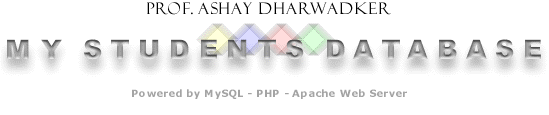| Home | Sign up! | Projects | Seminars | Research Notes | Today's Lecture | About |

Update Project Form
 Course: Project Topic: Project Description: Let X be a set together with a function  f : X  →  X. Given any x X, the iterates
f 1(x) =  f (x)
f 2(x) =  ff (x) )
f 3(x) =   fff (x) ) )
.
.
.
are all well-defined elements of X. We define the orbit of xX as the set {  f n(x) | n = 1,2,3,... }. In this context ( X, f ) is called a dynamical system. For example, the animation below shows the dynamical system (C, z 2 ).

The white points are initially inside a disc of radius 2 centered at the origin and 3 iterations are then performed. Some of the orbits tend to remain bounded while others tend to escape towards infinity.

Now let X= C ∪ {∞} be the extended complex plane and consider rational functions f (z) = p(z) | q(z) where p, q are polynomials without any common factors. The filled Julia set of the dynamical system ( C ∪ {∞}, p(z) | q(z) ) consists of all points whose orbits are bounded sets in the complex plane C. The Julia set of the dynamical system ( C ∪ {∞}, p(z) | q(z) ) is the boundary of the filled Julia set. A filled Julia set may be a connected set in the complex plane (aka Fatou region) or a totally disconnected set in the complex plane (aka Cantor dust).

We first consider the case f (z) = z 2 + c where c is a constant. It is a remarkable fact that the corresponding filled Julia sets are connected (i.e. form Fatou regions) if and only if c belongs to the Mandelbrot set. In fact, this can be taken as a definition of the Mandelbrot set, shown below in Figure 1.

The Mandelbrot set (white)
shown on [-1.5,0.5] × [-1,1]

Your Password: